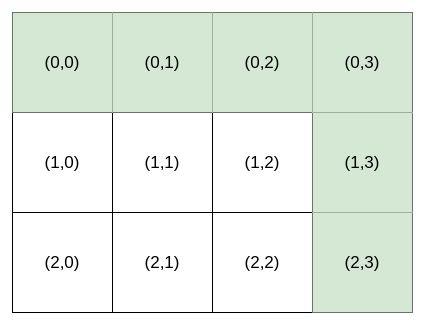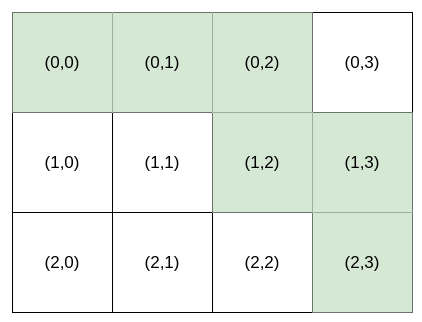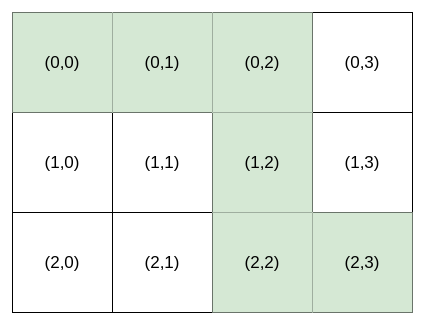Formatted question description: https://leetcode.ca/all/1643.html

# 1643. Kth Smallest Instructions (Hard)

Bob is standing at cell (0, 0), and he wants to reach destination: (row, column). He can only travel right and down. You are going to help Bob by providing instructions for him to reach destination.

The instructions are represented as a string, where each character is either:

• 'H', meaning move horizontally (go right), or
• 'V', meaning move vertically (go down).

Multiple instructions will lead Bob to destination. For example, if destination is (2, 3), both "HHHVV" and "HVHVH" are valid instructions.

However, Bob is very picky. Bob has a lucky number k, and he wants the kth lexicographically smallest instructions that will lead him to destination. k is 1-indexed.

Given an integer array destination and an integer k, return the kth lexicographically smallest instructions that will take Bob to destination.

Example 1:Input: destination = [2,3], k = 1
Output: "HHHVV"
Explanation: All the instructions that reach (2, 3) in lexicographic order are as follows:
["HHHVV", "HHVHV", "HHVVH", "HVHHV", "HVHVH", "HVVHH", "VHHHV", "VHHVH", "VHVHH", "VVHHH"].


Example 2:Input: destination = [2,3], k = 2
Output: "HHVHV"


Example 3:Input: destination = [2,3], k = 3
Output: "HHVVH"


Constraints:

• destination.length == 2
• 1 <= row, column <= 15
• 1 <= k <= nCr(row + column, row), where nCr(a, b) denotes a choose b​​​​​.

Related Topics:
Dynamic Programming

## Solution 1. Combination

Given destination: (row, column), we will have column H and row V in the result.

Let’s denote h = column, v = row meaning the number of H and V left to pick, respectively. The result is of length h + v.

We pick the character one by one for the result.

For the first character, if we pick H, the rest of characters can form c = nCr(h - 1 + v, v) combinations.

If k <= c, it means that the result is one of these c combinations. So we should pick H here. We append H to the result and --h.

Otherwise, we should pick V instead, and do --v and k -= c (skip these c combinations).

We keep doing this until we made choice for all the h + v characters.

// OJ: https://leetcode.com/problems/kth-smallest-instructions/

// Time: O((H + V)^2)
// Space: O(1)
class Solution {
int comb(int n, int r) {
long ans = 1;
for (int i = 1, j = n - r + 1; i <= r; ++i, ++j) ans = ans * j / i;
return ans;
}
public:
string kthSmallestPath(vector<int>& A, int k) {
int h = A, v = A, N = h + v;
string s;
for (int i = 0; i < N; ++i) {
if (h) { // we have H available to pick
int c = comb(h - 1 + v, v); // if we pick H at the current position, there will be c combinations for the rest of characters
if (k <= c) { // k is covered within c, so we should pick H.
s += 'H';
--h;
} else { // otherwise we should pick V
k -= c;
s += 'V';
--v;
}
} else { // no H left, have to pick V.
s += 'V';
--v;
}
}
return s;
}
};


Java

class Solution {
public String kthSmallestPath(int[] destination, int k) {
int rows = destination, columns = destination;
int length = rows + columns;
int[][] combinations = getCombinations(length);
StringBuffer sb = new StringBuffer();
for (int i = 0; i < length; i++) {
if (columns > 0) {
int count = combinations[rows + columns - 1][columns - 1];
if (k > count) {
k -= count;
sb.append('V');
rows--;
} else {
sb.append('H');
columns--;
}
} else {
sb.append('V');
rows--;
}
}
return sb.toString();
}

public int[][] getCombinations(int total) {
int[][] combinations = new int[total + 1][total + 1];
for (int i = 0; i <= total; i++)
combinations[i] = 1;
combinations = 1;
for (int i = 2; i <= total; i++) {
for (int j = 1; j <= i; j++)
combinations[i][j] = combinations[i - 1][j] + combinations[i - 1][j - 1];
}
return combinations;
}
}